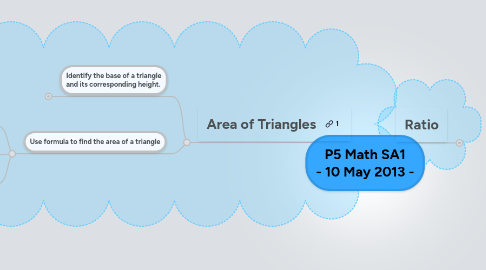# P5 Math SA1 - 10 May 2013 -

Get Started. It's FreeP5 Math SA1 - 10 May 2013 -## 1. Ratio

### 1.1. a : b

1.1.1. Order is important.

1.1.2. no units

1.1.3. Actual vs. Relative quantity

1.1.4. simplest form

1.1.4.1. Can both number be divided by:

1.1.4.1.1. 2 ?

1.1.4.1.2. The smaller of the two number?

1.1.4.1.3. 3 ?

1.1.5. Take note of the unit of measurement

1.1.5.1. Bag A is 1kg. Bag B is 500g. What is the ratio of Bag A to Bag B?

1.1.5.1.1. 1000 : 500 = 2 : 1

1.1.6. 'a' and 'b' are whole numbers.

1.1.6.1. 1 : 2

### 1.2. Equivalent Ratio

1.2.1. Find one quantity given the other quantity and the ratio of two quantities.

1.2.1.1. In 3 : 8 = 21 : g, what is the value represented by G?

1.2.2. Solve up to 2-step word problems involving ratios of two quantities.

1.2.2.1. Lenny and Stephy ate a box of sweets. The ratio of the number of sweets Lenny ate to the number of sweets Stephy ate is 5 : 8. If Stephy ate 40 sweets, how many sweets did Lenny eat?

### 1.3. a : b : c

1.3.1. Find one quantity given the other quantity and the ratio of three quantities.

1.3.1.1. 6 : 9 : 21 = A : 12 : B What are the values represented by A and B?

1.3.2. Solve up to 2-step word problems involving ratios of three quantities.

1.3.2.1. Aisyah used red, yellow and blue beads to make a necklace. The ratio of number of red beads to the number of yellow beads to the number of blue beads used was 9 : 4 : 7. If there was a total of 260 beads, how many more red than blue beads did she used?

### 1.4. Unitary Method of Problem Solving

1.4.1. Start and End of Question Stem

1.4.1.1. Mark and Sharon have some postcards in the ratio 2 : 3

1.4.1.2. 2/5 of the postcards belong to Mark and 3/5 of the postcards belong to Sharon.

1.4.1.3. 1/2 of Mark’s postcards is equal to 1/3 of Sharon’s postcards.

1.4.1.4. Mark has 2/3 as many postcards as Sharon.

1.4.2. Type of changes

1.4.2.1. One party unchanged

1.4.2.1.1. Sharon buys another 15 postcards [Mark remains unchanged]

1.4.2.2. Total unchanged

1.4.2.2.1. Mark gives 5 postcards to Sharon [total remains unchanged]

1.4.2.3. Difference unchanged

1.4.2.3.1. Mark and Sharon gives away 25 postcards each [difference remains unchanged]

1.4.2.3.2. Age Gap

## 2. Area of Triangles

### 2.1. Identify the base of a triangle and its corresponding height.

2.1.1. The breath of a triangle can be any of the sides of the triangle

2.1.2. The height of the triangle is a perpendicular line segment from the base to the opposite vertex of the triangle.

2.1.3. The height need not be inside the triangle.

### 2.2. Use formula to find the area of a triangle

2.2.1. Example 1:

2.2.2. Example 2:

2.2.3. Example 3: